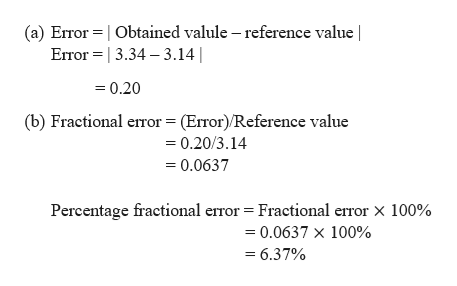# (4) A group of students measured the ratio of circumference to diameter to be 3.34. The reference value is 3.14. Determine the following:(a) Error  (b) Percentage fractional error

Question
6 views

(4) A group of students measured the ratio of circumference to diameter to be 3.34. The reference value is 3.14. Determine the following:

(a) Error

(b) Percentage fractional error

check_circle

Step 1

Given:

Student obtained the ratio of circumference and the diameter of the circle = 3.34

Reference value = 3.14

Step 2

Calculating the error and percentage frac...help_outlineImage Transcriptionclose(a) Error Obtained valule - reference value | 3.34 3.14 Error 0.20 (b) Fractional error (Error)/Reference value 0.20/3.14 0.0637 Percentage fractional error = Fractional error X 100% =0.0637 x 100% =6.37% fullscreen

### Want to see the full answer?

See Solution

#### Want to see this answer and more?

Solutions are written by subject experts who are available 24/7. Questions are typically answered within 1 hour.*

See Solution
*Response times may vary by subject and question.
Tagged in

### Units and Dimensions